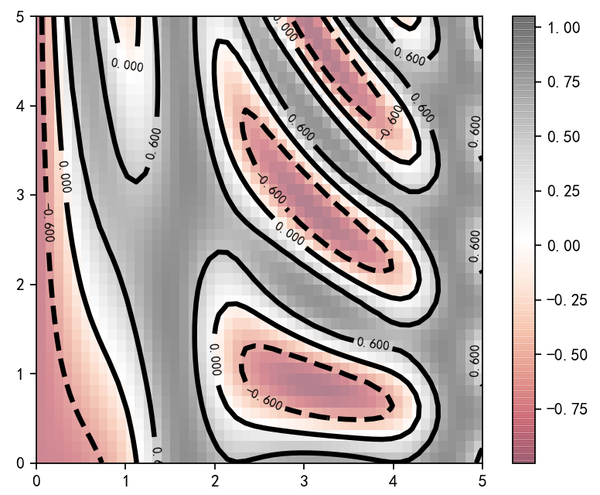# 等高线

• plt.contour: 绘制等高线
• plt.contourf: 绘制带有填充色的等高线
• plt.imshow: 显示图形
```def f(x, y):
return np.sin(x)**10 + np.cos(10 +y*x)*np.cos(x)

x = np.linspace(0,5,50)
y = np.linspace(0,5,40)

X, Y = np.meshgrid(x, y) # 使用两个一维数组构建一个二维数组
Z = f(X, Y)

plt.contour(X, Y, Z, colors='blue')
```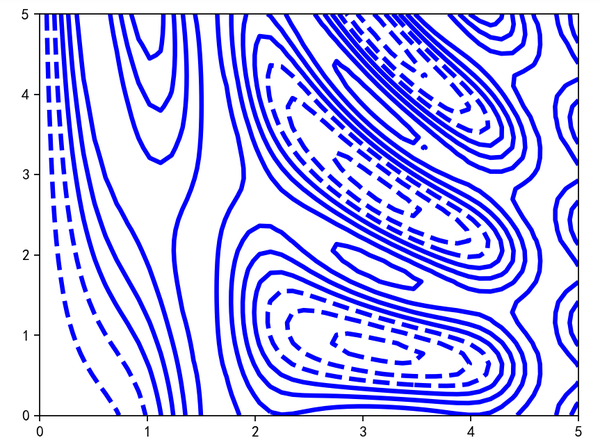contour函数需要三个基本参数：x、y、z轴三个坐标轴的网格数据。x与y轴表示图形中的位置，而z轴通过等高线的等级来表示。

```plt.contour(X, Y, Z, cmap='RdGy')
plt.colorbar()
```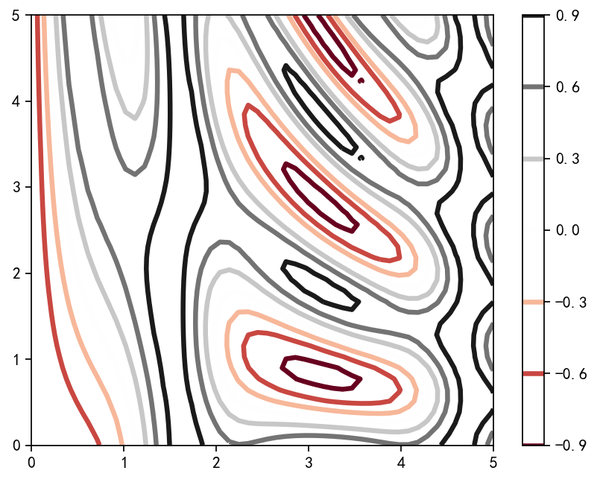`RdGy``红-灰`配色方案(Red-Gray)。Matplotlib有很多配色方案可选，都在plt.cm模块里，使用`plt.cm.<tab>`可以查看有哪些方案。

```plt.contourf(X, Y, Z, cmap='RdGy')
plt.colorbar()
```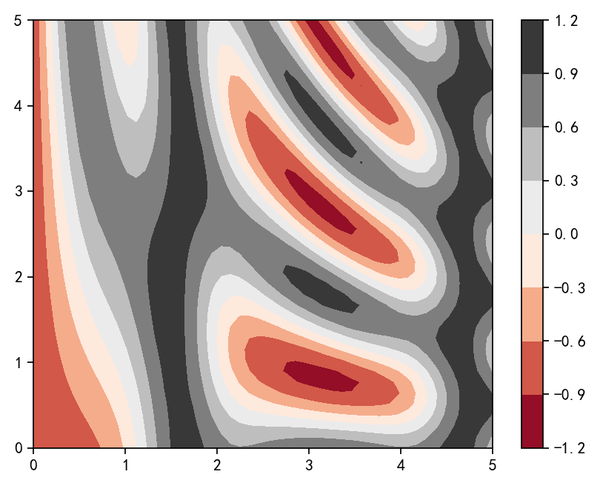```plt.imshow(Z, extent=[0,5,0,5], origin='lower',cmap='RdGy')
plt.colorbar()
plt.axis(aspect='image')
```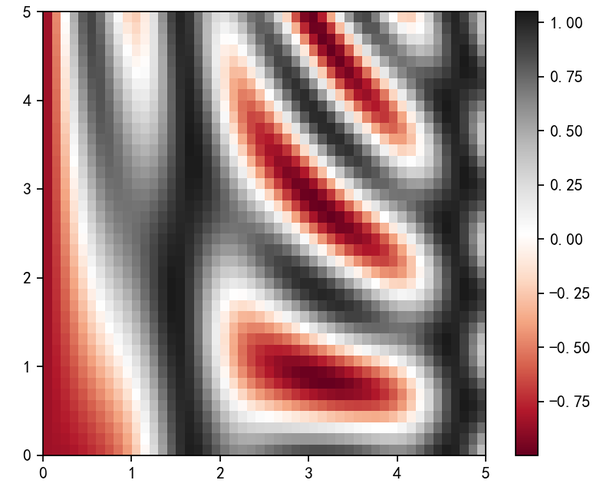• imshow不支持使用x和y轴的数据设置网格，必须通过extend参数设置图形的坐标范围`xmin、xmax，ymin、ymax`
• imshow默认使用标准的图形数组定义，原点位于左上角，类似浏览器。而不是绝大多数等高线的左下角
• imshow会自动调整坐标轴的精度以适应数据显示。可以通过`plt.axis(aspect='image')`来设置x与y轴的单位。

```contours = plt.contour(X,Y,Z,3,colors='black') # 分3级
plt.clabel(contours,inline=True, fontsize=8)  # 内部带数值文字

plt.imshow(Z, extent=[0,5,0,5], origin='lower',cmap='RdGy', alpha=0.5)
plt.colorbar()
```